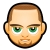## 哈，喷下面向对象与c 热

为什么80%的码农都做不了架构师？->>>＝＝＝＝＝＝＝＝＝＝＝＝ 如下＝＝＝＝＝＝＝＝＝＝＝＝＝＝＝

```typedef struct{
int x;
int y;
}_XY;
typedef struct{
int x;
int y;
int z;
}_XYZ;

#define _getX(p) (p)->x
#define _getY(p) (p)->y```

（注意，此处并不是说类似c++的面向对象语言的“类”设计方法论存在问题，纯粹的理论不存在对错，如果一个业务逻辑中处理的对象，本身具备类与类的关联和继承内容，采用这些“类”设计方法，会极大的提高编程实现效率，而如果它们并没有这些本质关联，仅仅是形式上可以如此表达，那么这种额外的形式化设计，只会把简单点事情复杂化）。

```typedef struct{
int x;
int y;
}_2D;
typedef struct{
_2D xy;
}_XY;
typedef struct{
_2D xy;
int z;
}_XYZ;```

```_XY d2;
_XYZ d3;
#define _copy2D(p1,p2) do{_getX(p1) = _getX(p2);_getY(p1) = _getY(p2);}while (0)

_copy2D(d2->xy,d3->xy);```

```typedef struct{
int x;
int y;
}_XY;
typedef struct{
int z;
int y;
}_YZ;
typedef struct{
int z;
int x;
}_XZ;
typedef struct{
int x;
int y;
int z;
}_XYZ;

#define _getX(p) (p)->x
#define _getY(p) (p)->y
#define _getZ(p) (p)->z
#define _copyXY(p1,p2) do{_getX(p1) = _getX(p2);_getY(p1) = _getY(p2);}while (0)
#define _copyYZ(p1,p2) do{_getZ(p1) = _getZ(p2);_getY(p1) = _getY(p2);}while (0)
#define _copyXZ(p1,p2) do{_getZ(p1) = _getZ(p2);_getX(p1) = _getX(p2);}while (0)```

```#define _copy2D(p1,p2,_get1,_get2) do{_get1(p1) = _get1(p2);_get2(p1) = _get2(p2);}while (0)
#define _copyXY(p1,p2) _copy2D(p1,p2,_getX,_getY)
#define _copyYZ(p1,p2) _copy2D(p1,p2,_getZ,_getY)
#define _copyXZ(p1,p2) _copy2D(p1,p2,_getX,_getZ)```

_copy2D：将两个数据的两个对应维度的数据进行复制。后者复制给前者。
_copyXY: 将两个数据指定的x,y维度进行复制
_copyYZ，_copyXZ等同描述。

#define _getX(p) (p)->x

#define _chkX(p) (_getX(p))

```#define _Inext(pI,i) ((pI) + i)->_next
#define _Iprec(pI,i) ((pI) + i)->_prec```

```#define _travell(pI,cur,op,...) do{\
_I __cur = cur;\
do {\
op(__VA_ARGS__);\
__cur = _Inext(pI,__cur);\
}while(__cur != cur);\
}while (0)```

＝＝＝＝＝＝＝＝＝＝文稿部分内容结束＝＝＝＝＝＝＝

0
m

_XY和_XYZ可以有共同的超类，一种可以投影到OXY平面上的点，因而存在共同的操作：计算出在OXY平面上的坐标getXY()。

0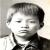#### 引用来自“magiclogy”的评论

_XY和_XYZ可以有共同的超类，一种可以投影到OXY平面上的点，因而存在共同的操作：计算出在OXY平面上的坐标getXY()。

0

0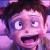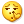看到哈字，我就知道这个文章是中山野鬼写的，哈
0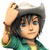@中山野鬼 ，出书吧。你出本C相关的书我绝对买正版。我最近正想强攻C。@Liuxd 加快写完吧。一直在努力，不过能力有限，没法一天就是几万字的那么潇洒，对应代码倒是早就好了。。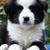C的经典书一大堆了。野鬼的文笔不理想，估计你买了也是吃灰。
0